Concepts

Class 10
Chapter 12 Class 10 - Electricity

## Graphical Representation Volt and Amperes

Experiment to Verify Ohm's Law

We take a conductor (Example Nichrome Wire)

We connect it to a circuit containing Voltmeter and Ammeter

When we supply current, we measure reading of Potential Difference with the help of Voltmeter and Electric Current with help of Ammeter

We calculate Ratio of Potential Difference/Electric Current

Now,we increase amount of current,

We again measure reading of Potential Difference and Electric Current and again Calculate Ratio

We note that Ratio Remains the Same

Hence Ohm's Law, which states that Ratio of Potential Difference and Electric Current Remains the same, is verified

## Q1. The values of Current (I) flowing through a conductor for the corresponding values of potential difference (V) are given. Plot a graph between V and I.

 V (Volt) 2.5 5 10 15 25 I (Ampere) 0.1 0.2 0.4 0.6 1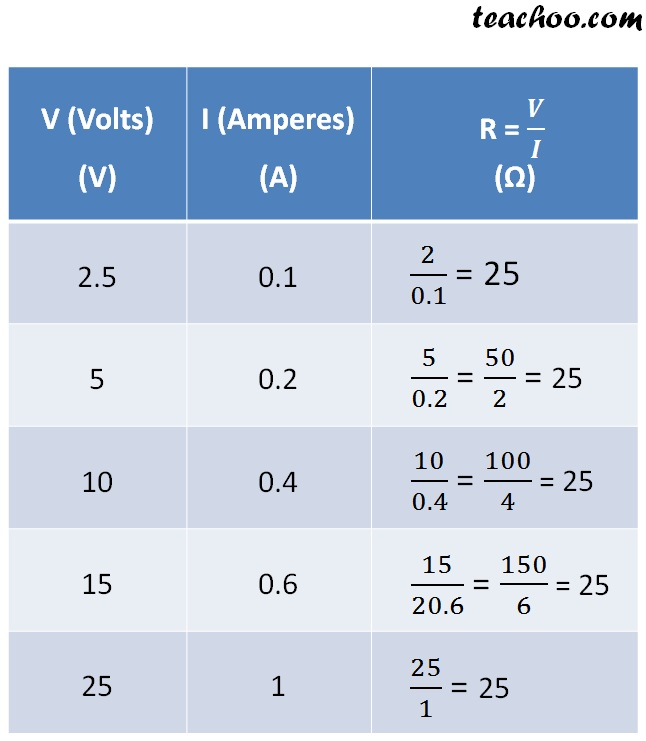From the above table

We can see that,

the ratio of 𝑉/𝐼 is always constant.

This gives resistance

The resistance is 25 in above case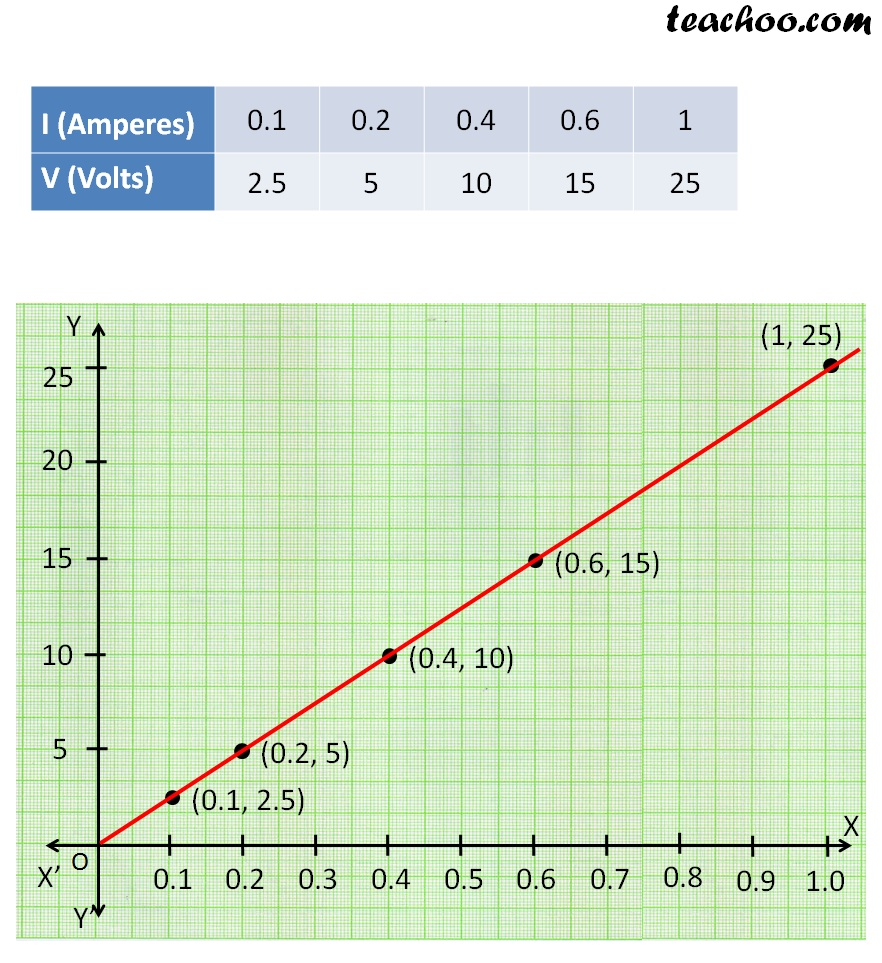## Q2. The values of Current (I) flowing through a conductor for the corresponding values of potential difference (V) are given. Plot a graph between V and I.

 I (Ampere) 0.5 1 2 2.5 3 V (Volt) 2 4 8 10 12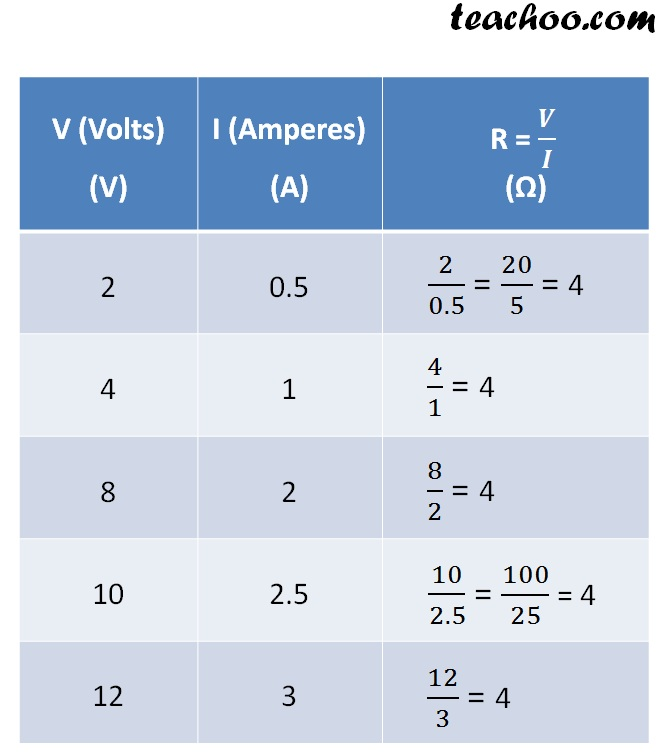From the above table

We can see that,

the ratio of V/I is always  constant.

This gives resistance

The resistance is 4 in above case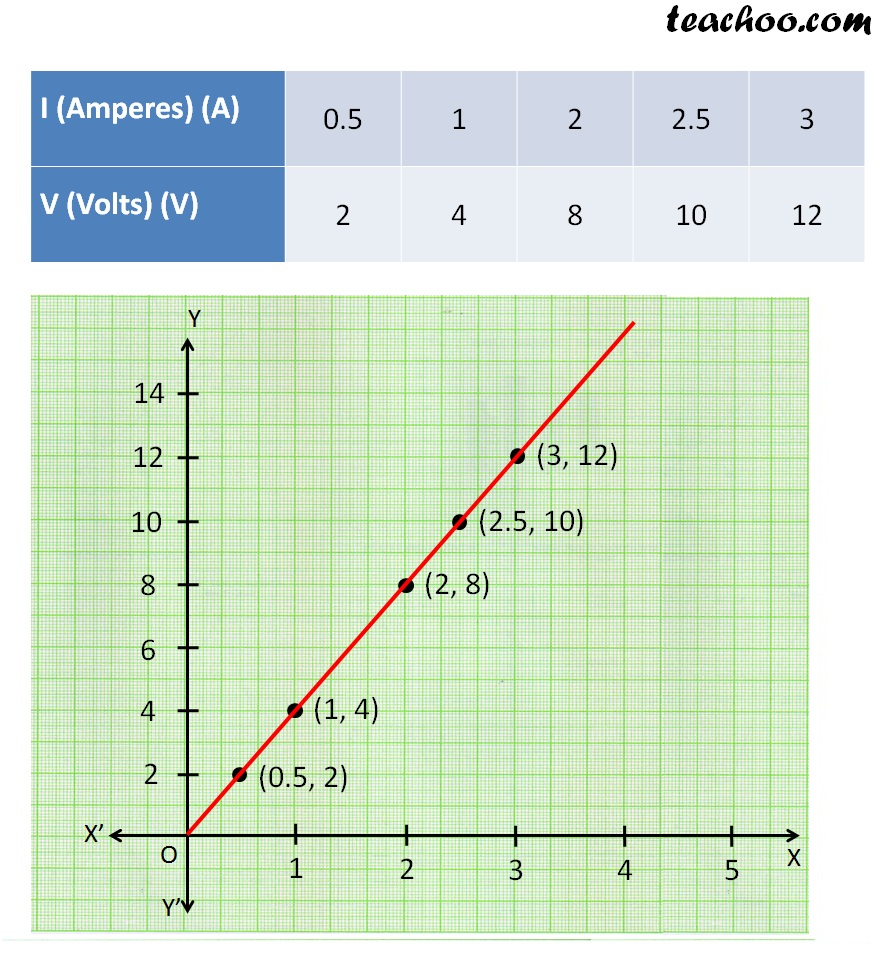## Q3. The values of Current (I) flowing through a conductor for the corresponding values of potential difference (V) are given. Plot a graph between V and I. Hence. find the resistance.

 I (Ampere) 0.5 1 2 3 4 V (Volt) 1.6 3.3 6.7 10.2 13.2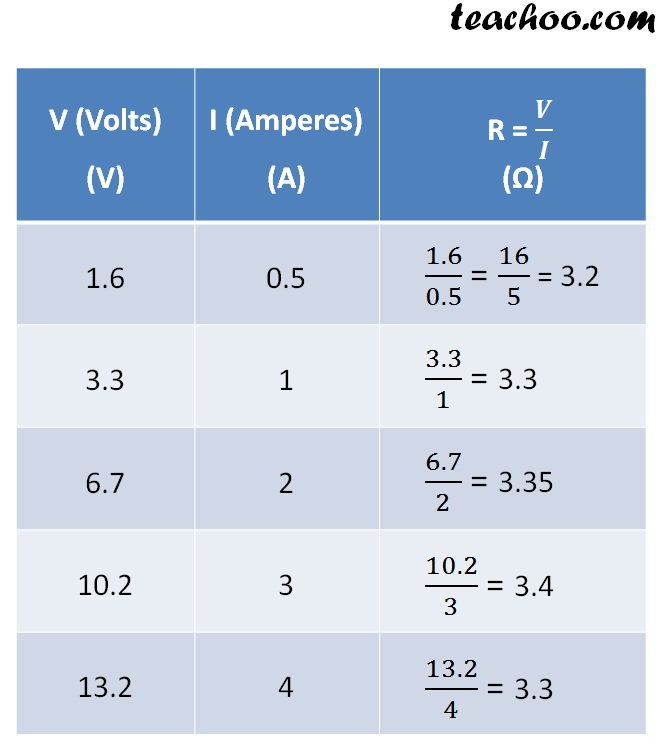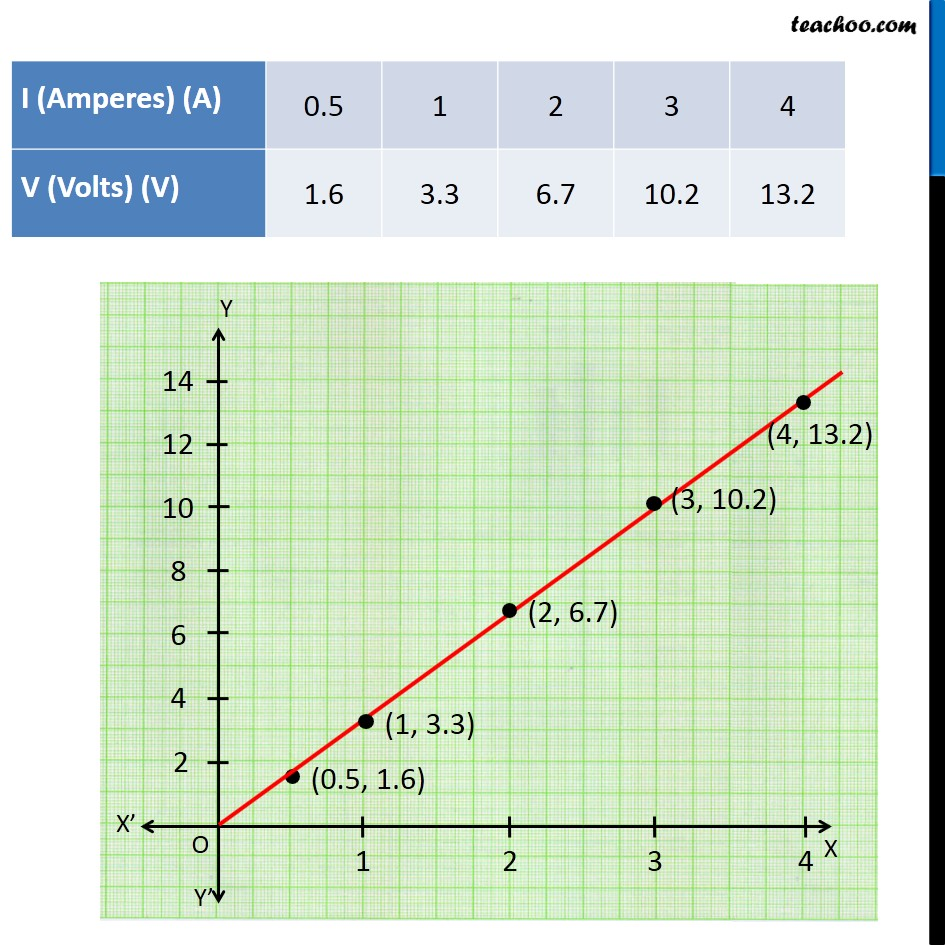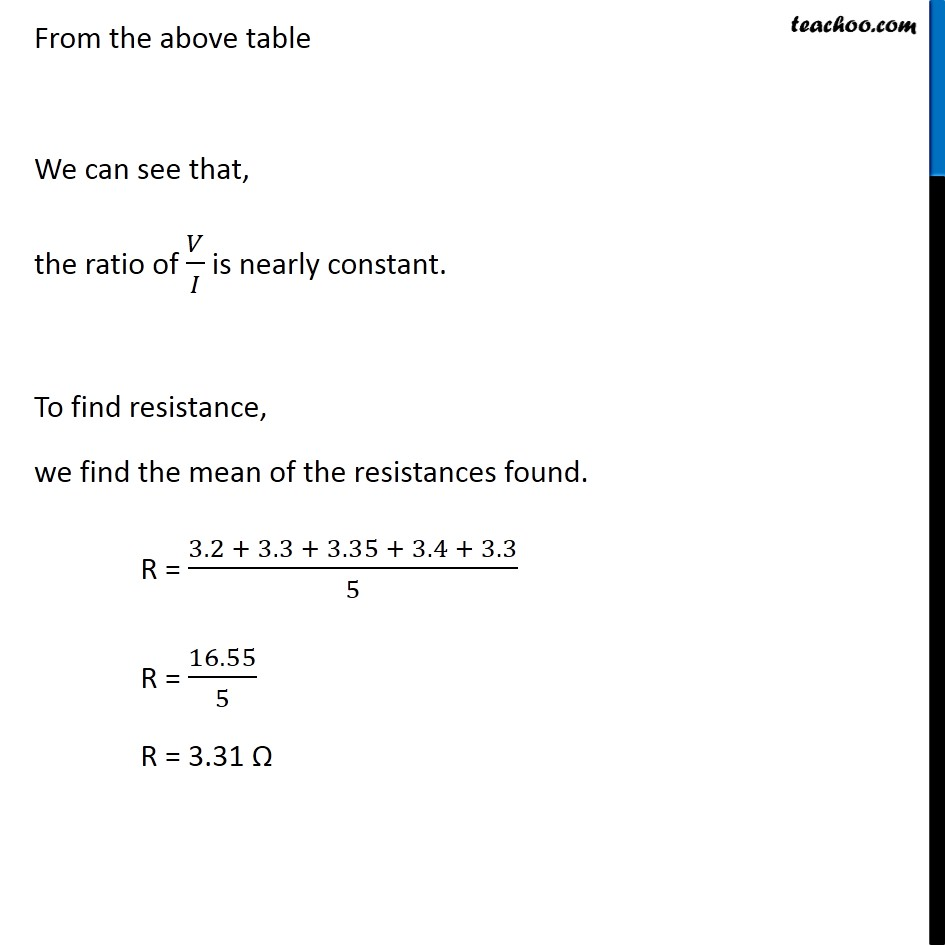Learn in your speed, with individual attention - Teachoo Maths 1-on-1 Class

### Transcript

From the above table We can see that, the ratio of 𝑉/𝐼 is nearly constant. To find resistance, we find the mean of the resistances found. R = (3.2 + 3. 3 + 3.35 + 3.4 + 3.3)/5 R = 16.55/5 R = 3.31 Ω